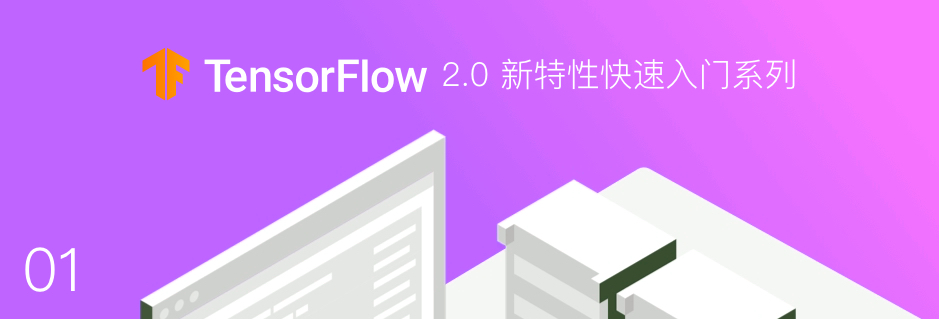## TensorFlow 2.0 新增变化特性TensorFlow 2.0 版本现已推出，相对于 1.x 版本在很多地方都有较大的变动。文章中，我们将了解并学习 TensorFlow 2.0 的一些新的特征，同时熟悉一些常用的新 API 方法。

### TensorFlow 2.0 安装

2019 年初，TensorFlow 官方推出了 2.0 预览版本，也意味着 TensorFlow 即将从 1.x 过度到 2.x 时代。• 使用 Keras 和 Eager Execution 轻松构建模型。
• 在任意平台上实现稳健的生产环境模型部署。
• 为研究提供强大的实验工具。
• 通过清理废弃的 API 和减少重复来简化 API。

pip uninstall tensorflow -y  # 卸载 TensorFlow 1.x
pip install tf-nightly-2.0-preview  # 安装 TensorFlow 2.0

import tensorflow as tf

tf.__version__

    '2.0.0-dev20190401'

### Eager Execution

TensorFlow 2.0 带来的最大改变之一是将 1.x 的 Graph Execution（图与会话机制）更改为 Eager Execution（动态图机制）。在 1.x 版本中，低级别 TensorFlow API 首先需要定义数据流图，然后再创建 TensorFlow 会话，这一点在 2.0 中被完全舍弃。

TensorFlow 2.0 中的 Eager Execution 是一种命令式编程环境，可立即评估操作，无需构建图：操作会返回具体的值，而不是构建以后再运行的计算图。实际上，Eager Execution 在 1.x 的后期版本中也存在，但需要单独执行 tf.enable_eager_execution() 进行手动启用。不过，2.0 版本的 TensorFlow 默认采用了 Eager Execution，无法关闭并回到 1.x 的 Graph Execution 模式中。

1.x 版本中，如果我们新建一个张量 tf.Variable([[1, 2], [3, 4]]) 并执行输出，那么只能看到这个张量的形状和属性，并不能直接输出其数值。结果如下：

<tf.Variable 'Variable:0' shape=(2, 2) dtype=int32_ref>

c = tf.Variable([[1, 2], [3, 4]])
c

    <tf.Variable 'Variable:0' shape=(2, 2) dtype=int32, numpy=
array([[1, 2],
[3, 4]], dtype=int32)>

c.numpy()

    array([[1, 2],
[3, 4]], dtype=int32)

Eager Execution 带来的好处是不再需要手动管理图和会话。例如，现在使用示例张量进行数学计算，可以像 Python 一样直接相加。

c + c  # 加法计算

    <tf.Tensor: id=14, shape=(2, 2), dtype=int32, numpy=
array([[2, 4],
[6, 8]], dtype=int32)>

init_op = tf.global_variables_initializer()  # 初始化全局变量
with tf.Session() as sess:  # 启动会话
sess.run(init_op)
print(sess.run(c + c))  # 执行计算


Eager Execution 带来的好处显而易见，其进一步降低了 TensorFlow 的入门门槛。之前，因为图与会话的模式，让很多人在入门时都很纳闷。除此之外，得益于自动微分的使用，在 Eager Execution 期间，可以使用 tf.GradientTape 这类跟踪操作以便稍后计算梯度。

w = tf.Variable([[1.0]])  # 新建张量

with tf.GradientTape() as tape:  # 追踪梯度
loss = w * w


    <tf.Tensor: id=31, shape=(1, 1), dtype=float32, numpy=array([[2.]], dtype=float32)>

tf.GradientTape 会像磁带一样记录下计算图中的梯度信息，然后使用 .gradient 即可回溯计算出任意梯度，这对于使用 TensorFlow 低阶 API 构建神经网络时更新参数非常重要。

Eager Execution 的好处很多，但带来的问题也是很明显的，尤其是对于已经熟练使用 TensorFlow 的工程师而言简直是噩梦。

### TensorFlow Keras

TensorFlow 1.x 中，我们可以通过 tf.layers 高阶层封装开快速搭建神经网络。如果，2.0 已完全移除了 tf.layers 模块，转而引入了 tf.keras

### 其他变化

TensorFlow 2.0 带来的更多变化，请详细阅读 官方说明。除此之外，官方也已经更新 TensorFlow 2.0 版本的 API 文档